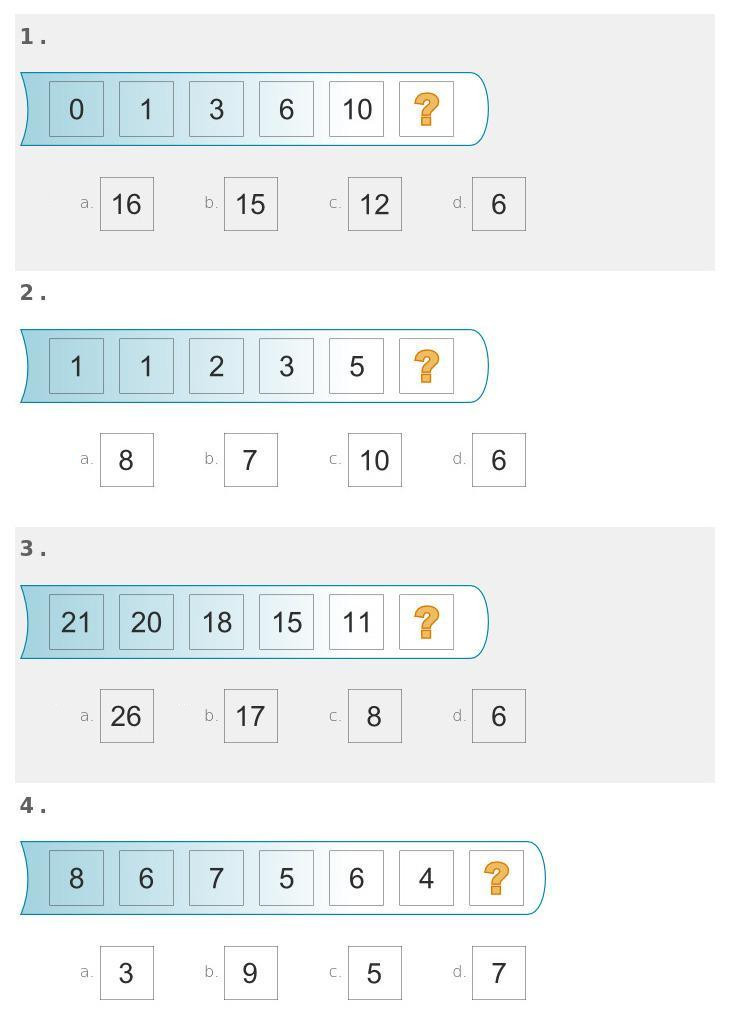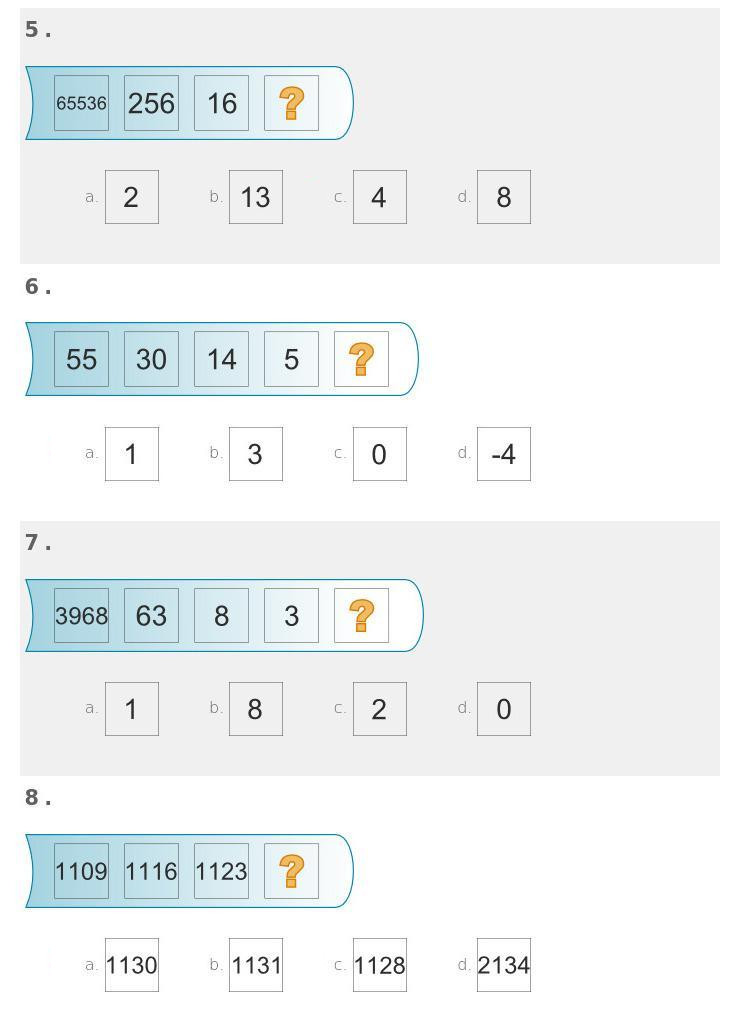# Numerical IQ Tests

In the first lecture we looked at sequences of numbers and used derivatives to predict the future. Predicting the future of some outcome is part what one calls intelligence. Once gains insight into a situation and predicts the outcome. One has tried to measure intelligence quotients (IQ's) with various ways. Whether they measure intelligence is really questionable but IQ tests are interesting for various reasons. First of all they can easily be hacked by learning. They do therefore not measure IQ but knowledge. The question ``What is intelligence" is interesting. Whatever we have used to define it has quickly been overtaken by artificial intelligence. A computer has absolutely no problem with numerical IQ tests as before.
In the following test, there is a numerical reasoning test. You are given a sequence and you have to find the next one:
```Sequence                       Choice
---------------------------------------------------
0,1,3,6,10                     16,15,12,6
1,1,2,3,5                      8,7,10,6
21,20,18,15,11                 26,17,8,6
8,6,7,5,6,4                    3,9,5,7
65536,256,16                   2,13,4,8
55,30,14,5                     1,3,0,-4
3968,63,8,3                    1,8,2,0
1109,1116,1123                 1130,1131,1128,2134
```
Most of the questions can be solved by calculus. There are some, where the iteration rule is super exponential like 16,256,65536, where one squares the previous term or then 3,8,63,3968, where the pattern is to square and subtract one. Still, what it all boils down is to look at differences or differences of logarithms or differences of log of logs. For example, the pattern of the sequence 65536,256,16 is seen after taking twice the log to the base 2 (which is the inverse of exp1(x) = 2x).
```Log[2,Log[2,{65536,256,16}]]
```
gives {4,3,2} which obviously continuous as 1, so that the next number is 221=4. The test writers (and most solvers) probably do it directly and see that 256 is the square root of 65536 and that 16 is the square root of 256. It still is a calculus idea.